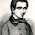## Thursday, August 31, 2017

### Looking for new quantum axioms

Philip Ball writes a Quanta mag essay:
The Flimsy Foundations of Quantum Mechanics ...

Scientists have been using quantum theory for almost a century now, but embarrassingly they still don’t know what it means.
Lubos Motl adequately trashes it as an anti-quantum crackpot article, and I will not attempt to outdo his rant. I agree with him.

Instead, I focus on one fallacy at the heart of modern theoretical physics. Under this fallacy, the ideal theory is one that is logically derived from postulates, and where one can have a metaphysical belief in those postulates independent of messy experiments.

Ball writes:
But this so-called rule for calculating probabilities was really just an intuitive guess by the German physicist Max Born. So was Schrödinger’s equation itself. Neither was supported by rigorous derivation. Quantum mechanics seems largely built of arbitrary rules like this, some of them — such as the mathematical properties of operators that correspond to observable properties of the system — rather arcane. It’s a complex framework, but it’s also an ad hoc patchwork, lacking any obvious physical interpretation or justification.

Compare this with the ground rules, or axioms, of Einstein’s theory of special relativity, which was as revolutionary in its way as quantum mechanics. (Einstein launched them both, rather miraculously, in 1905.) Before Einstein, there was an untidy collection of equations to describe how light behaves from the point of view of a moving observer. Einstein dispelled the mathematical fog with two simple and intuitive principles: that the speed of light is constant, and that the laws of physics are the same for two observers moving at constant speed relative to one another. Grant these basic principles, and the rest of the theory follows. Not only are the axioms simple, but we can see at once what they mean in physical terms.

What are the analogous statements for quantum mechanics?
Ball is wrong on many levels.

Quantum mechanics does have a simple set of axioms like special relativity. See the Dirac–von Neumann axioms:
In mathematical physics, the Dirac–von Neumann axioms give a mathematical formulation of quantum mechanics in terms of operators on a Hilbert space. They were introduced by Dirac (1930) and von Neumann (1932).
Einstein did not really tidy up the equations for light. He did not simplify Maxwell's equations at all, in that 1905 paper.

Poincare's long 1905 paper did that. He wrote them in 4-vector form on spacetime, and proved that they were covariant with respect to the Lorentz transformation group. He gave a 4D action formula for electromagnetism, thereby giving an alternate proof of covariance. Einstein did none of that, and did not even understand it until maybe ten years later, if ever. Later H. Weyl and others explained that electromagnetism is just the field theory you get from an external circle symmetry group.

The real problem with Ball's essay is what infects everyone in quantum gravity, string theory, black hole information, multiverse, and other such fields. It is a belief that all of physics must be derived from metaphysical principles or axioms, without real-world experiments. It is the modern equivalent of medieval theologians wanting to deduce everything from the Bible.

Physics has always advanced from experiments, and not from metaphysical/axiomatic thinking. The proponents of the latter approach always point to Einstein's 1905 special relativity paper, but their historical analysis is always wrong.

My book, How Einstein Ruined Physics, details all of this.

#### 1 comment:

1.Evy Galois completely ignored physics for a reason: it's an infinite regress. lol